# Estimating moments with Monte Carlo¶

Let us denote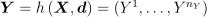, where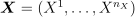is a random vector, and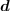a deterministic vector. We seek here to evaluate, the characteristics of the central part (central tendency and spread i.e. mean and variance) of the probability distribution of a variable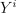, using the probability distribution of the random vector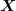.

The Monte Carlo method is a numerical integration method using sampling, which can be used, for example, to determine the mean and standard deviation of a random variable(if these quantities exist, which is not the case for all probability distributions):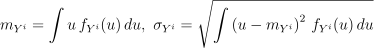where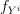represents the probability density function of.

Suppose now that we have the sample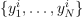of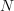values randomly and independently sampled from the probability distribution; this sample can be obtained by drawing asample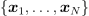of the random vector(the distribution of which is known) and by computing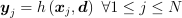. Then, the Monte-Carlo estimations for the mean and standard deviation are the empirical mean and standard deviations of the sample: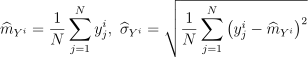These are just estimations, but by the law of large numbers their convergence to the real values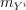and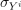is assured as the sample sizetends to infinity. The Central Limit Theorem enables the difference between the estimated value and the sought value to be controlled by means of a confidence interval (especially ifis sufficiently large, typically> a few dozens even if there is now way to say for sure if the asymptotic behavior is reached). For a probabilitystrictly between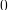and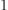chosen by the user, one can, for example, be sure with a confidence, that the true value ofis between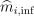and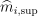calculated analytically from simple formulae. To illustrate, for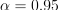: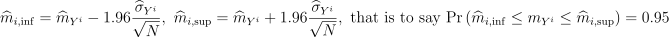The size of the confidence interval, which represents the uncertainty of this mean estimation, decreases asincreases but more gradually (the rate is proportional to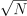: multiplyingby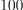reduces the length of the confidence interval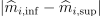by a factor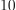).

This method is also referred to as Direct sampling, crude Monte Carlo method, Classical Monte Carlo integration.